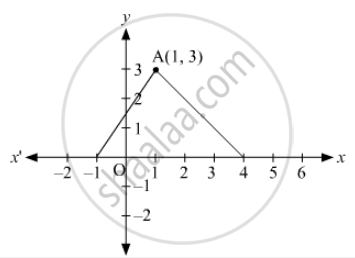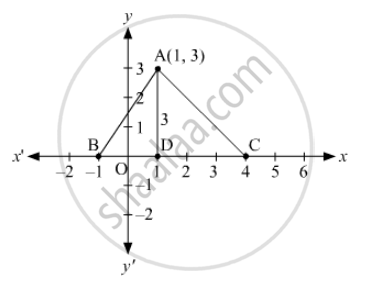Advertisement Remove all ads

# In Fig. 14.46, the Area of δAbc (In Square Units) is - Mathematics

MCQ

In Fig. 14.46, the area of ΔABC (in square units) is#### Options

• 15

• 10

• 7.5

• 2.5

Advertisement Remove all ads

#### SolutionThe coordinates of A are (1, 3).
∴ Distance of A from the x-axis, AD = y-coordinate of A = 3 units
The number of units between B and C on the x-axis are 5.
∴ BC = 5 units
Now,
Area of ∆ABC = $\frac{1}{2} \times BC \times AD = \frac{1}{2} \times 5 \times 3 = \frac{15}{2} = 7 . 5$  square units

Thus, the area of ∆ABC is 7.5 square units.

Is there an error in this question or solution?
Advertisement Remove all ads

#### APPEARS IN

RD Sharma Class 10 Maths
Chapter 6 Co-Ordinate Geometry
Q 43 | Page 66
Advertisement Remove all ads

#### Video TutorialsVIEW ALL 

Advertisement Remove all ads
Share
Notifications

View all notifications

Forgot password?